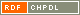Naslov: On the connectivity of bipartite distance-balanced graphs Miklavič, Štefko (Avtor)Šparl, Primož (Avtor)http://dx.doi.org/10.1016/j.ejc.2011.10.002 Angleški jezik Delo ni kategorizirano 1.01 - Izvirni znanstveni članek IAM - Inštitut Andrej Marušič A connected graph ▫$\varGamma$▫ is said to be distance-balanced whenever for any pair of adjacent vertices ▫$u,v$▫ of ▫$\varGamma$▫ the number of vertices closer to ▫$u$▫ than to ▫$v$▫ is equal to the number of vertices closer to ▫$v$▫ than to ▫$u$▫. In [K. Handa, Bipartite graphs with balanced ▫$(a,b)$▫-partitions, Ars Combin. 51 (1999), 113-119] Handa asked whether every bipartite distance-balanced graph, that is not a cycle, is 3-connected. In this paper the Handa question is answered in the negative. Moreover, we show that a minimal bipartite distance-balanced graph, that is not a cycle and is not 3-connected, has 18 vertices and is unique. In addition, we give a complete classification of non-3-connected bipartite distance-balanced graphs for which the minimal distance between two vertices in a 2-cut is three. All such graphs are regular and for each ▫$k \geq 3$▫ there exists an infinite family of such graphs which are ▫$k$▫-regular.Furthermore, we determine a number of structural properties that a bipartite distance-balanced graph, which is not 3-connected, must have. As an application, we give a positive answer to the Handa question for the subfamily of bipartite strongly distance-balanced graphs. graph theory, connected graphs, connectivity, distance-balanced graphs, bipartite graphs 2012 str. 237-247 Vol. 33, no. 2 0195-6698 519.17 10243697481280 45Gradivo ni uvrščeno v področja.

Skupna ocena: (0 glasov) Ocenjevanje je dovoljeno samo prijavljenim uporabnikom.Postavite miškin kazalec na naslov za izpis povzetka. Klik na naslov izpiše podrobnosti ali sproži prenos.

## Sekundarni jezik

Jezik: Slovenski jezik teorija grafov, povezani grafi, povzanost, razdaljno uravnoteženi grafi, dvodelni grafi

## Komentarji

Dodaj komentar

izpis_zaKomentiranje

Komentarji (0)
 0 - 0 / 0 Ni komentarjev!

Nazaj